# yyaxis

Create chart with two y-axes

## Syntax

``yyaxis left``
``yyaxis right``
``yyaxis(ax,___)``

## Description

example

````yyaxis left` activates the side of the current axes associated with the left y-axis. Subsequent graphics commands target the left side. If the current axes do not include two y-axes, then this command adds a second y-axis. If there are no axes, then this command first creates them.```
````yyaxis right` activates the side of the current axes associated with the right y-axis. Subsequent graphics commands target the right side.```

example

````yyaxis(ax,___)` specifies the active side for the axes `ax` instead of the current axes. If the axes do not include two y-axes, then this command adds a second y-axis. Specify the axes as the first input argument. Use single quotes around `'left'` and `'right'`.```

## Examples

collapse all

Create axes with a y-axis on both the left and right sides. Plot a set of data against the left y-axis. Then, use `yyaxis right` to activate the right side so that subsequent graphics functions target it. Plot a second set of data against the right y-axis and set the limits for the right y-axis.

```x = linspace(0,10); y = sin(3*x); yyaxis left plot(x,y) z = sin(3*x).*exp(0.5*x); yyaxis right plot(x,z) ylim([-150 150])```Create a chart with two y-axes and add a title and axis labels to each side.

Load the matrix `hwydata` from the example file `accidents.mat`. Create a scatter plot of the fifth column in `hwydata` against the left y-axis. Add a title and axis labels.

```load('accidents.mat','hwydata') ind = 1:51; drivers = hwydata(:,5); yyaxis left scatter(ind,drivers) title('Highway Data') xlabel('States') ylabel('Licensed Drivers (thousands)')```Create a second scatter plot of the seventh column in `hwydata` against the right y-axis. Then, label the right y-axis.

```pop = hwydata(:,7); yyaxis right scatter(ind,pop) ylabel('Vehicle Miles Traveled (millions)')```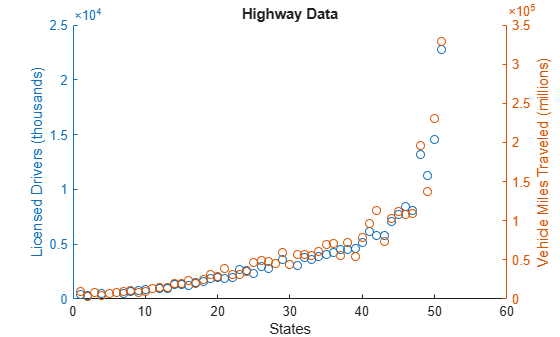Plot two lines against the left y-axis by using the `hold on` command.

```x = linspace(0,10); yl1 = sin(x); yl2 = sin(x/2); yyaxis left plot(x,yl1) hold on plot(x,yl2) ```Plot two lines against the right y-axis. The `hold` command affects both the left and right y-axes, so you do not need to reissue it. After plotting, turn `hold` back off.

```yr1 = x; yr2 = x.^2; yyaxis right plot(x,yr1) plot(x,yr2) hold off ```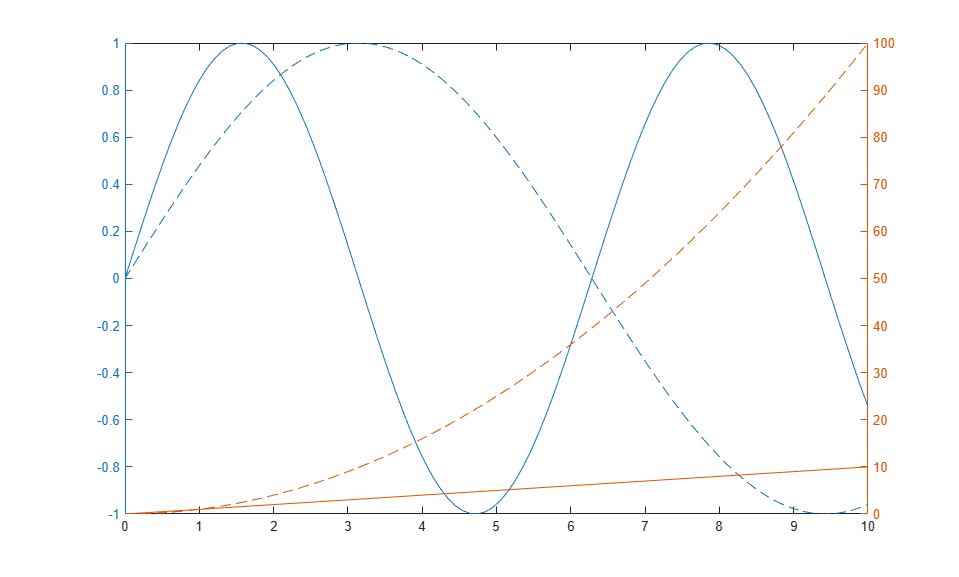Clear the left side by making it active and then using the `cla` command.

```yyaxis left cla ```Specify the color scheme for each side of the axes by setting the color order to the two colors that you want to use. Starting in R2019b, you can use the `colororder` function to set the color order. Then, plot two lines against the left y-axis and two lines against the right y-axis. Add a legend.

```colororder({'b','m'}) yyaxis left y = [1 2; 3 4]; plot(y) yyaxis right z = [4 3; 2 1]; plot(z) legend```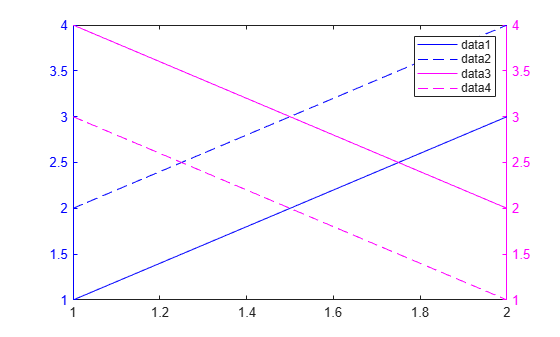Control individual plot colors by setting the color order for each side of the axes.

Plot three bar charts against the left side. Use a different color for each bar series by setting the color order for the left side to the default color order.

```yyaxis left bar(magic(3)); colororder('default')```

Plot three scatter plots against the right side. Use a different color for each scatter plot by setting the color order to an array of color names. Alternatively, you can specify the colors using a matrix of RBG triplets. Then add a legend.

```yyaxis right scatter([1 2 3],[2 5 2],'filled') hold on scatter([1 2 3],[3 4 1],'filled') scatter([1 2 3],[4 2 4],'filled') hold off colororder({'r','b','c'}) legend```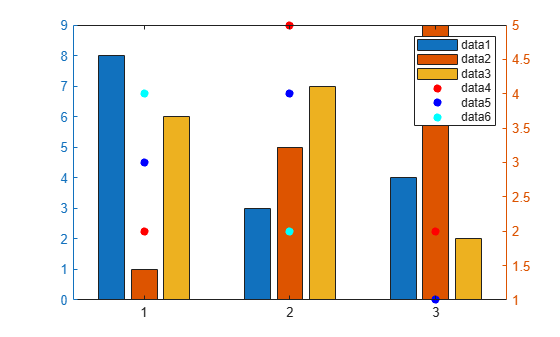Starting in R2019b, you can display a tiling of plots using the `tiledlayout` and `nexttile` functions. Call the `tiledlayout` function to create a 2-by-1 tiled chart layout. Call the `nexttile` function to create the axes objects `ax1` and `ax2`. Add a second y-axis to the top axes by specifying `ax1` as the first input to `yyaxis`. If you do not specify the axes, then `yyaxis` adds a second y-axis to the current axes.

```x = linspace(1,10); tiledlayout(2,1) % Top plot ax1 = nexttile; yyaxis(ax1,'left') plot(ax1,x,sin(x)) yyaxis(ax1,'right') plot(ax1,x,exp(x)) % Bottom plot ax2 = nexttile; plot(ax2,1:10)```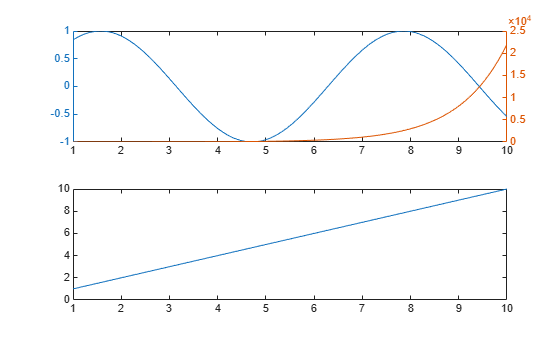## Input Arguments

collapse all

Target axes, specified as an `Axes` object. If you do not specify an `Axes` object, then `yyaxis` uses the current axes.

## Limitations

• When working with two y-axes, you cannot:

## Tips

• To determine which side of the axes is active, query the `YAxisLocation` property for the `Axes` object. The property is set to `'left'` when the left side is active and `'right'` when the right side is active. The `YAxisLocation` property for an `Axes` object with two y-axes is read only.

• To clear the active side, use `cla`. To clear both sides of the axes and remove the right y-axis, use `cla reset`. Alternatively, you can wait to clear both sides and remove the right y-axis until the next plotting command by setting the `NextPlot` property of the `Axes` object to `'replaceall'`.

• The `Children` property of the `Axes` object only contains the children for the active side. To access all the children for both sides, use the `allchild` function.

## Algorithms

collapse all

### Grid Lines

Grid lines correspond with the tick mark locations along the left y-axis.

### Colors and Line Styles

Plots associated with a particular side of the axes use the same color as the y-axis on that side. If a side contains multiple lines, then the lines cycle through the line style order. The left y-axis uses the first color in the color order of the `Axes` object, and the right y-axis uses the second color.

If you add a second y-axis to an `Axes` object that contains charts, then the existing charts and the left y-axis do not change colors. The right y-axis uses the next color in the color order.

### Axes Properties

Axes properties related to the y-axis have two values. However, MATLAB® gives access only the value for the active side. For example, if the left side is active, then the `YLim` property of the `Axes` object contains the limits for the left y-axis. However, if the right side is active, then the `YLim` property contains the limits for the right y-axis.

An exception is that the `YAxis` property of the `Axes` object contains an array of two ruler objects (one for each y-axis). You can use the rulers to access each y-axis without depending on the active side. For an example, see Modify Properties of Charts with Two y-Axes.